Author: Oscar Cronquist Article last updated on February 16, 2018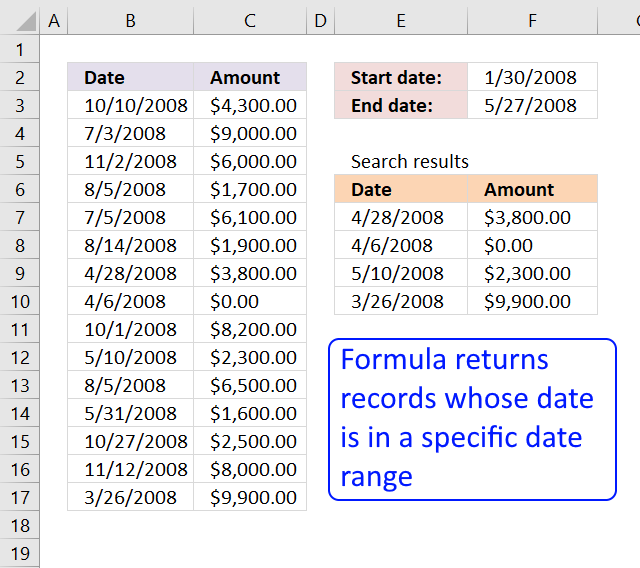Question: How to filter rows using dates?

Answer:

In this post I will describe how to:

### Filter rows using array formulas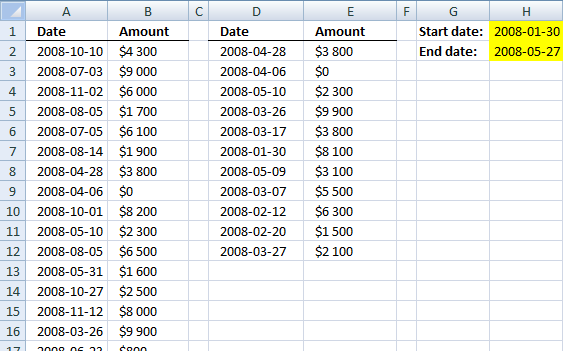Array formula in cell D2:

=INDEX(\$A\$2:\$B\$38, SMALL(IF((\$A\$2:\$A\$38=\$H\$1), MATCH(ROW(\$A\$2:\$A\$38), ROW(\$A\$2:\$A\$38)), ""), ROW(A1)), COLUMN(A1))

How to create an array formula

1. Select cell D2
2. Type the array formula
3. Press and hold Ctrl + Shift
4. Press Enter once
5. Release all keys

How to copy array formula

1. Select cell D2
2. Copy cell D2 (Ctrl + c)
3. Select cell D2:E9
4. Paste (Ctrl + v)
How to remove #num errors
=IFERROR(INDEX(\$A\$2:\$B\$38, SMALL(IF((A2:\$A\$38<\$H\$2)*(A2:\$A\$38>\$H\$1), MATCH(ROW(A2:\$A\$38), ROW(A2:\$A\$38)), ""), ROW(A1)), COLUMN(A1)),"")

Download excel sample file for this tutorial.

filter-records-between-two-dates.xls
(Excel 97-2003 Workbook *.xls)

### Filter rows using an excel table

Convert range to a table

1. Select range A2:B38
2. Go to tab "Insert"
3. Click "Table"
4. Click OK!

Filter dates

1. Click Black arrow near Date header
2. Hover over "Date filters"
3. Click "Between..."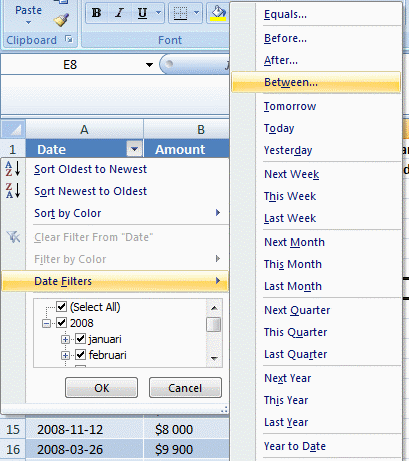4. Select dates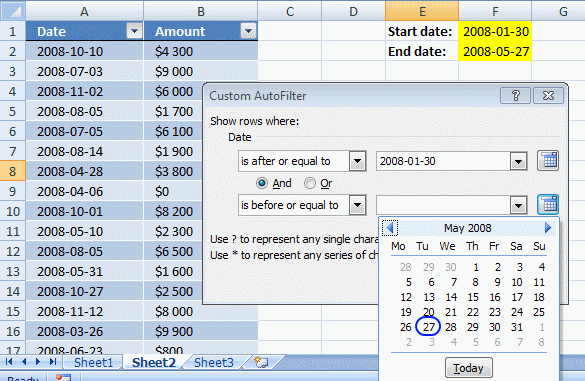5. Click OK!

### Filter rows using excel table and vba

In this example, you can type a date in cell B1 and B2. Press the button and the table is instantly filtered. Copy the vba code below into a standard module. Create a button and assign the macro.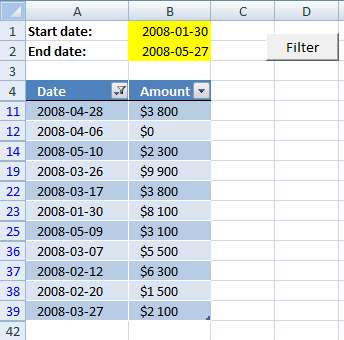VBA code

```Sub TblFilterDates()

Worksheets("Sheet3").ListObjects("Table13").Range.AutoFilter _
Field:=1, Criteria1:=">=" & Worksheets("Sheet3").Range("B1") _
, Operator:=xlAnd, Criteria2:="<=" & Worksheets("Sheet3").Range("B2")
End Sub```

Where to copy vba code?

1. Press Alt+F11
2. Insert a new module
3. Copy paste code into code window
How to create a button
1. Click "Developer" tab
2. Click "Insert" button
3. Create a button (form control)
4. Right click on the button
5. Assign your macro

Download excel sample file for this tutorial.

filter-records-between-two-dates.xlsm
(Excel 2007 MacroEnabled Workbook *.xlsm)